• 目录 语法 说明 例子 1.在当前坐标区上添加图例 2.在特定坐标区上添加图例 ...legend函数是在坐标区上添加图例。 语法 legend legend(label1,...,labelN) legend(labels) legend(subset,___) legend(tar...
目录
语法
说明
例子
1.在当前坐标区上添加图例
2.在特定坐标区上添加图例
3.在执行绘图命令的过程中指定图例标签
4.图例位置和列数
5.在分块图布局中显示共享图例
6.在图例中包含部分图形对象
7.为图例添加标题
8.删除图例背景
9.修改图例外观

legend函数是在坐标区上添加图例。
语法
legend
legend(label1,...,labelN)
legend(labels)
legend(subset,___)
legend(target,___)
legend(___,'Location',lcn)
legend(___,'Orientation',ornt)
legend(___,Name,Value)
legend(bkgd)
lgd = legend(___)
legend(vsbl)
legend('off')
说明
   legend 为每个绘制的数据序列创建一个带有描述性标签的图例。对于标签，图例使用数据序列的 DisplayName 属性中的文本。如果 DisplayName 属性为空，则图例使用 'dataN' 形式的标签。当在坐标区上添加或删除数据序列时，图例会自动更新。此命令为 gca 返回的当前坐标区或图形创建图例。如果当前坐标区为空，则图例为空。如果坐标区不存在，此命令将创建坐标区。
legend(label1,...labelN) 设置图例标签。以字符向量或字符串列表形式指定标签，例如 legend('Jan','Feb','Mar')。
   legend(labels) 使用字符向量元胞数组、字符串数组或字符矩阵设置标签，例如 legend({'Jan','Feb','Mar'})。
   legend(subset,___) 仅在图例中包括 subset 中列出的数据序列的项。subset 以图形对象向量的形式指定。您可以在指定标签之前或不指定其他输入参数的情况下指定 subset。
   legend(target,___) 使用由 target 指定的坐标区或图，而不是当前坐标区或图。指定 target 作为第一个输入参数。
   legend(___,'Location',lcn) 设置图例位置。例如，'Location','northeast' 将在坐标区的右上角放置图例。请在其他输入参数之后指定位置。
   legend(___,'Orientation',ornt)（其中 ornt 为 'horizontal'）并排显示图例项。ornt 的默认值为 'vertical'，即垂直堆叠图例项。
   legend(___,Name,Value) 使用一个或多个名称-值对组参数来设置图例属性。
   legend(bkgd)（其中 bkgd 为 'boxoff'）删除图例背景和轮廓。bkgd 的默认值为 'boxon'，即显示图例背景和轮廓。
   lgd = legend(___) 返回 Legend 对象。可使用 lgd 在创建图例后查询和设置图例属性。有关属性列表，可参考legend属性。
   legend(vsb1) 控制图例的可见性，其中 vsbl 为 'hide'、'show' 或 'toggle'。
   legend('off') 删除图例。
例子
1.在当前坐标区上添加图例
绘制两个线条并在当前坐标区上添加一个图例。将图例标签指定为legend函数的输入参数。
x = linspace(0,pi);
y1 = cos(x);
plot(x,y1)

hold on
y2 = cos(2*x);
plot(x,y2)

legend('cos(x)','cos(2x)')
如图所示：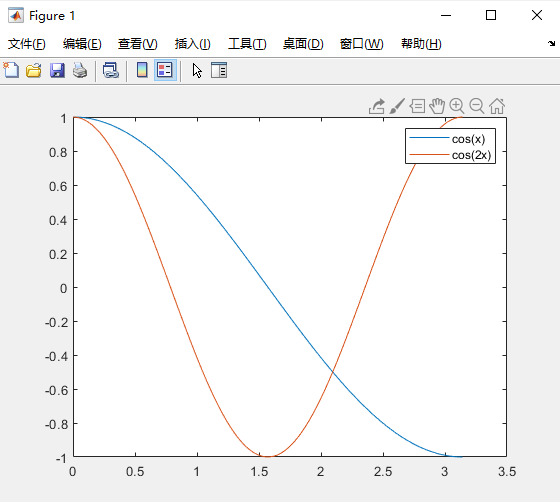如果在坐标区添加或删除数据序列，图例会相应地更新。创建数据序列时，可通过将 DisplayName 属性设置为名称-值对组来控制新数据序列的标签。如果不指定标签，则图例使用 'dataN' 形式的标签。

注意：如果您不希望在坐标区中添加或删除数据序列时自动更新图例，可将图例的 AutoUpdate 属性设置为 'off'。

x = linspace(0,pi);
y1 = cos(x);
plot(x,y1)

hold on
y2 = cos(2*x);
plot(x,y2)

legend('cos(x)','cos(2x)')

y3 = cos(3*x);
plot(x,y3,'DisplayName','cos(3x)')
hold off
如图所示：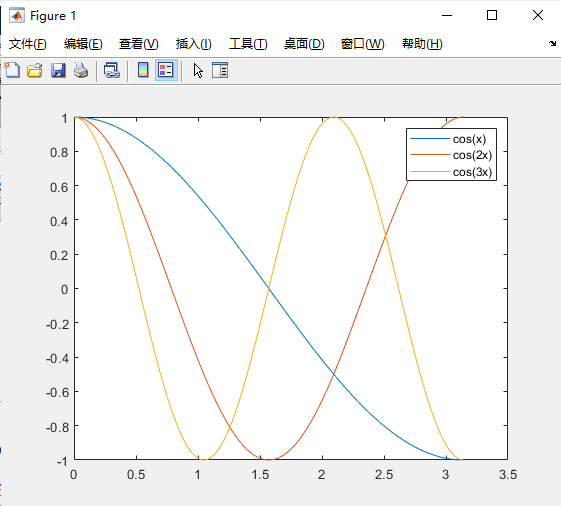删除图例。如图所示：
legend('off')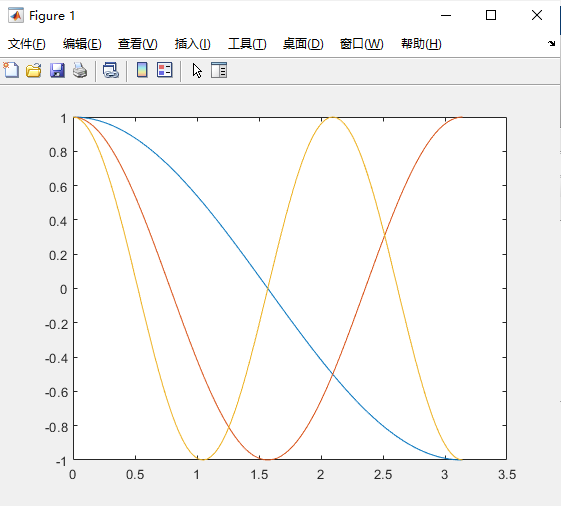2.在特定坐标区上添加图例
从R2019b开始，可以使用tiledlayout和nexttile函数显示分块图。调用tiledlayout函数以创建一个 2×1 分块图布局。调用nexttile函数以创建坐标区对象ax1和 ax2。在每个坐标区中对随机数据绘图。通过指定ax1作为 legend的第一个输入参数，在上部绘图中添加一个图例。
tiledlayout(2,1)
y1 = rand(3);
ax1 = nexttile;
plot(y1)

y2 = rand(5);
ax2 = nexttile;
plot(y2)

legend(ax1,{'Line 1','Line 2','Line 3'})
3.在执行绘图命令的过程中指定图例标签
绘制两个线条。通过将 DisplayName 属性设置为所需的文本，在执行绘图命令的过程中指定图例标签。然后，添加一个图例。
x = linspace(0,pi);
y1 = cos(x);
plot(x,y1,'DisplayName','cos(x)')

hold on
y2 = cos(2*x);
plot(x,y2,'DisplayName','cos(2x)')
hold off

legend
如图所示：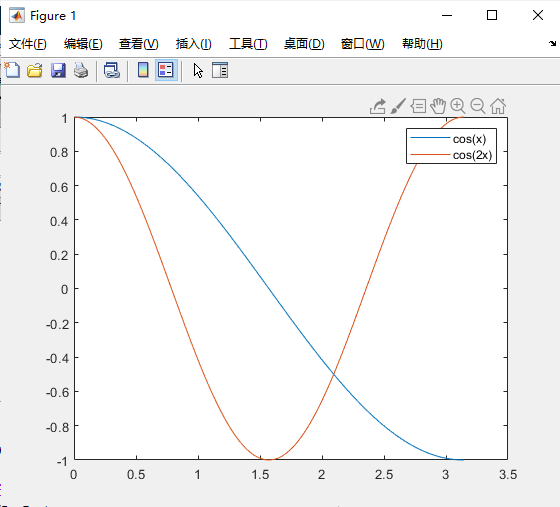4.图例位置和列数
绘制四个线条。在坐标区的西北角创建一个图例。使用NumColumns属性指定图例的列数。
x = linspace(0,pi);
y1 = cos(x);
plot(x,y1)

hold on
y2 = cos(2*x);
plot(x,y2)

y3 = cos(3*x);
plot(x,y3)

y4 = cos(4*x);
plot(x,y4)
hold off

legend({'cos(x)','cos(2x)','cos(3x)','cos(4x)'},'Location','northwest','NumColumns',2)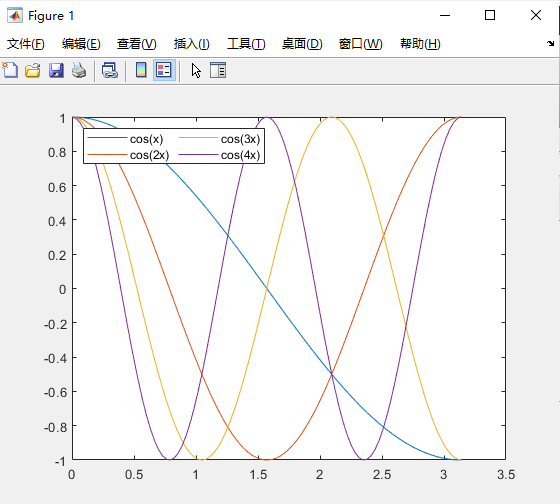默认情况下，图例项逐列从上到下排列。要逐行从左到右排列图例项，请将 Orientation 属性设置为 'horizontal'。
5.在分块图布局中显示共享图例
当要在两个或多个图之间共享一个图例时，可以在布局的一个单独图块中显示该图例。
在一个分块图布局中创建三个绘图。
tiledlayout(2,2);
nexttile
plot(rand(5))
nexttile
plot(rand(5))
nexttile
plot(rand(5))
添加一个共享图例，并将其移至第四个图块。
tiledlayout(2,2);
nexttile
plot(rand(5))
nexttile
plot(rand(5))
nexttile
plot(rand(5))

lgd = legend;
lgd.Layout.Tile = 4;
6.在图例中包含部分图形对象
如果不想将绘制的所有图形对象都包含在图例中，可以指定要包含的图形对象。
绘制三个线条并返回创建的 Line 对象。创建只包含其中两条线的图例。将第一个输入参数指定为要包含的 Line 对象的向量。
x = linspace(0,pi);
y1 = cos(x);
p1 = plot(x,y1);

hold on
y2 = cos(2*x);
p2 = plot(x,y2);

y3 = cos(3*x);
p3 = plot(x,y3);
hold off

legend([p1 p3],{'First','Third'})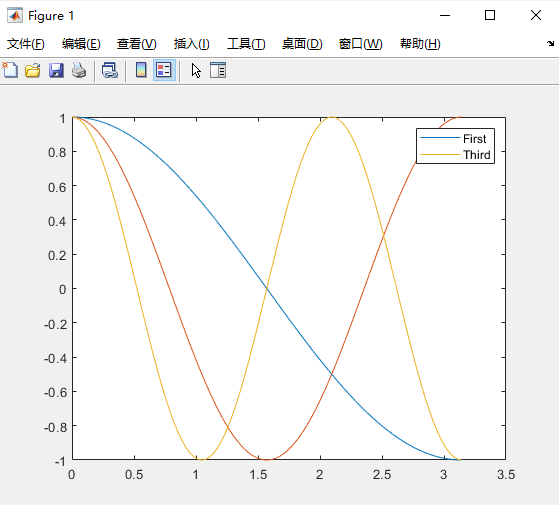7.为图例添加标题
绘制两个线条并创建一个图例。然后为图例添加标题。
x = linspace(0,pi);
y1 = cos(x);
plot(x,y1)

hold on
y2 = cos(2*x);
plot(x,y2)
hold off

lgd = legend('cos(x)','cos(2x)');
title(lgd,'My Legend Title')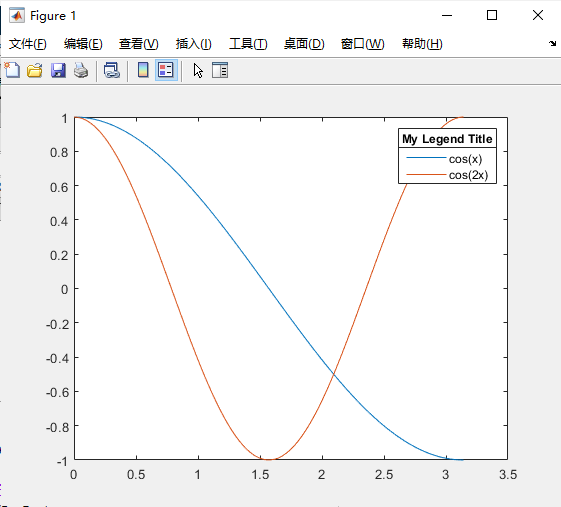8.删除图例背景
绘制两个线条并在坐标区左下角创建一个图例。然后，删除图例的背景和轮廓。
x = linspace(0,pi);
y1 = cos(x);
plot(x,y1)

hold on
y2 = cos(2*x);
plot(x,y2)
hold off

legend({'cos(x)','cos(2x)'},'Location','southwest')
legend('boxoff')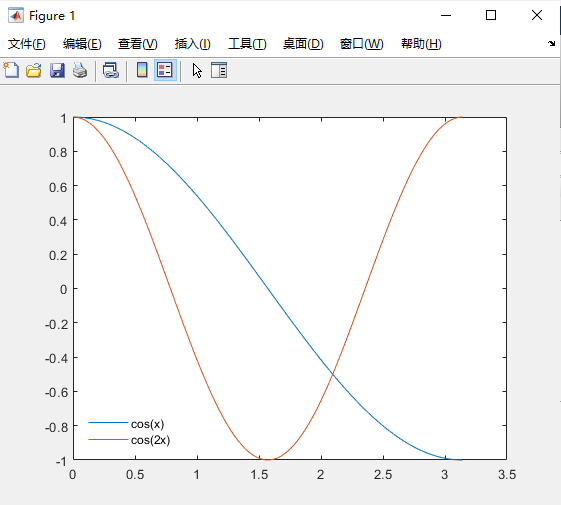9.修改图例外观
通过设置Legend属性来修改图例外观。创建图例时，可以在 legend 命令中使用名称-值对组来设置属性。还可以在创建图例后使用 Legend 对象来设置属性。
绘制四行随机数据。创建图例并将 Legend 对象赋给变量 lgd。使用名称-值对组设置 FontSize 和 TextColor 属性。
rdm = rand(4);
plot(rdm)

lgd = legend({'Line 1','Line 2','Line 3','Line 4'},'FontSize',12,'TextColor','blue')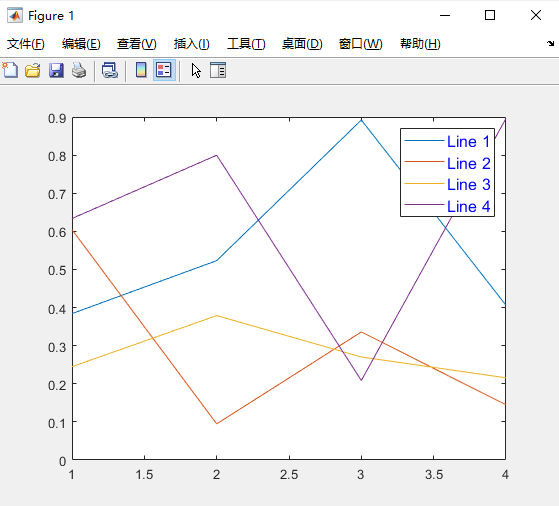lgd =
Legend (Line 1, Line 2, Line 3, Line 4) with properties:

String: {'Line 1'  'Line 2'  'Line 3'  'Line 4'}
Location: 'northeast'
Orientation: 'vertical'
FontSize: 12
Position: [0.7088 0.7034 0.1778 0.1957]
Units: 'normalized'

Show all properties

创建图例后，通过引用lgd来修改图例。使用对象圆点属性名称表示法设置NumColumns属性。
lgd.NumColumns = 2;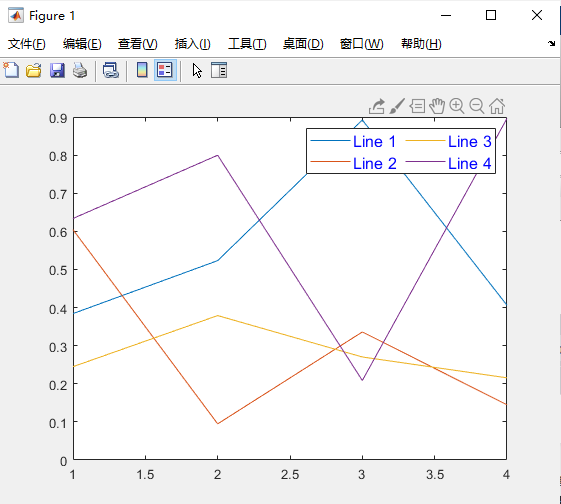展开全文• ## R语言中Legend函数的参数详解

万次阅读 多人点赞 2018-03-09 13:43:52
legend(x, y = NULL, legend, fill = NULL, col = par("col"), border = "black", lty, lwd, pch, angle = 45, density = NULL, bty = "o", bg = par("bg"), box.lwd = ...
legend(x, y = NULL, legend, fill = NULL, col = par("col"),

border = "black", lty, lwd, pch,
angle = 45, density = NULL, bty = "o", bg = par("bg"),
box.lwd = par("lwd"), box.lty = par("lty"), box.col = par("fg"),
pt.bg = NA, cex = 1, pt.cex = cex, pt.lwd = lwd,
xjust = 0, yjust = 1, x.intersp = 1, y.intersp = 1,
adj = c(0, 0.5), text.width = NULL, text.col = par("col"),
text.font = NULL, merge = do.lines && has.pch, trace = FALSE,
plot = TRUE, ncol = 1, horiz = FALSE, title = NULL,
inset = 0, xpd, title.col = text.col, title.adj = 0.5,
seg.len = 2)

x, yX,y用于定位图例，也可用单键词"bottomright", "bottom", "bottomleft", "left", "topleft", "top", "topright", "right" and "center"legend字符或表达式向量fill用特定的颜色进行填充col图例中出现的点或线的颜色border当fill = 参数存在的情况下，填充色的边框lty, lwd图例中线的类型与宽度pch点的类型angle阴影的角度density阴影线的密度bty图例框是否画出，o为画出，默认为n不画出bgbty != "n"时，图例的背景色box.lty, box.lwd, box.colbty = "o"时，图例框的类型，box.lty决定是否为虚线，box.lwd决定粗线，box.col决定颜色pt.bg点的背景色cex字符大小pt.cex点的大小pt.lwd点的边缘的线宽x.intersp图例中文字离图片的水平距离y.intersp图例中文字离图片的垂直距离adj图例中字体的相对位置text.width图例字体所占的宽度text.col图例字体的颜色text.font图例字体mergelogical, if TRUE，合并点与线，但不填充图例框，默认为TRUEtracelogical; if TRUE显示图例信息.plotlogical. If FALSE不画出图例ncol图例中分类的列数horizlogical; if TRUE,水平放置图例title给图例加标题inset当图例用关键词设置位置后，inset = 分数，可以设置其相对位置xpdxpd=FALSE，即不允许在作图区域外作图，改为TRUE即可，与par()参数配合使用。title.col标题颜色title.adj图例标题的相对位置，0.5为默认，在中间。0最左，1为最右。seg.lenlty 与lwd的线长，长度单位为字符宽度
展开全文• plt.legend()函数的作用是给图像加图例。 图例是集中于地图一角或一侧的地图上各种符号和颜色所代表内容与指标的说明，有助于更好的认识地图。 官方手册对该函数的功能进行了详细的介绍 博主对matplotlib中其他函数...
plt.legend()函数的作用是给图像加图例。 图例是集中于地图一角或一侧的地图上各种符号和颜色所代表内容与指标的说明，有助于更好的认识地图。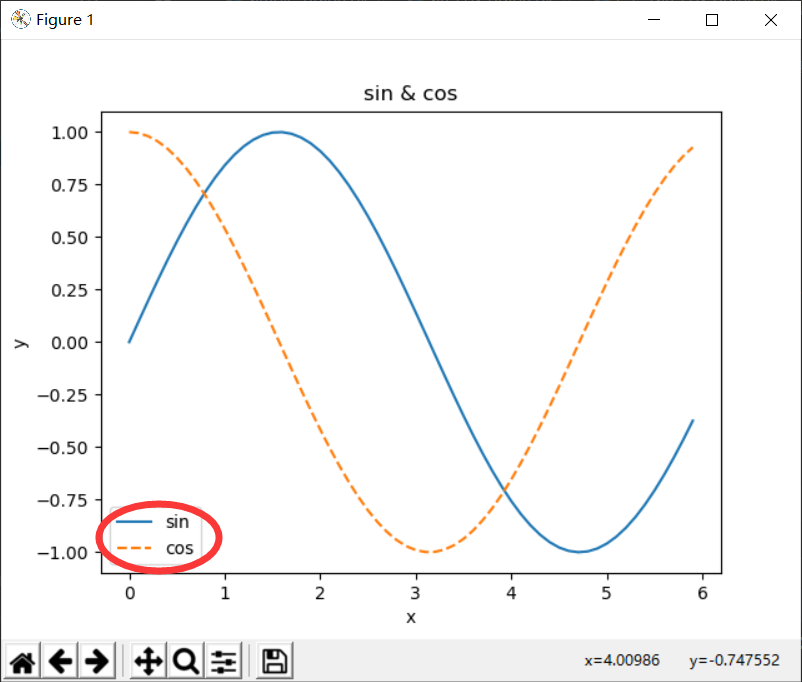官方手册对该函数的功能进行了详细的介绍 博主对matplotlib中其他函数也有介绍 下面对其中常用的一些功能做简单汇总： 1、设置图例位置：plt.legend(loc=‘xxx’)
location stringlocation code‘best’0‘upper right’1‘upper left’2‘lower left’3‘lower right’4‘right’5‘center left’6‘center right’7‘lower center’8‘‘upper center’’9‘center’10
2、设置图例字体大小 fontsize ：int or float or {‘xx-small’, ‘x-small’, ‘small’, ‘medium’, ‘large’, ‘x-large’, ‘xx-large’} 图例的字体大小。 如果该值为数字，则大小将是绝对字体大小（以磅为单位）。若是字符串，则相当于当前默认字体大小。仅当未指定prop时才使用此参数。
3、设置图例边框和背景 plt.legend(loc=‘best’,frameon=False) #去掉图例边框 plt.legend(loc=‘best’,edgecolor=‘blue’) #设置图例边框颜色 plt.legend(loc=‘best’,facecolor=‘blue’) #设置图例背景颜色,若无边框,参数无效
4、设置图例标题plt.legend(title=‘xxx’) plt.legend(title=(‘sinx’, ‘cosx’))则图例如图：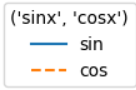5、设置图例与线条对应关系 可以先在绘制过程中设置label，这样直接在图例中会直接显示线条名词。
# coding: utf-8
import numpy as np
import matplotlib.pyplot as plt

# 生成数据
x = np.arange(0, 6, 0.1) # 以0.1为单位，生成0到6的数据
y1 = np.sin(x)
y2 = np.cos(x)

# 绘制图形
plt.plot(x, y1, label='sin')
plt.plot(x, y2, linestyle="--", label='cos')
plt.xlabel("x")  # x轴的标签
plt.ylabel("y")  # y轴的标签
plt.title('sin & cos')
plt.legend()
plt.show()

这样绘制出的图例如图：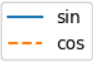展开全文• legend(x, y = NULL, legend, fill = NULL, col = par("col"), border = "black", lty, lwd, pch, angle = 45, density = NULL, bty = "o", bg = par("bg"), box.lwd = par("lwd"), box.lty = par("lty"), box....
legend(x, y = NULL, legend, fill = NULL, col = par("col"),
border = "black", lty, lwd, pch,
angle = 45, density = NULL, bty = "o", bg = par("bg"),
box.lwd = par("lwd"), box.lty = par("lty"), box.col = par("fg"),
pt.bg = NA, cex = 1, pt.cex = cex, pt.lwd = lwd,
xjust = 0, yjust = 1, x.intersp = 1, y.intersp = 1,
adj = c(0, 0.5), text.width = NULL, text.col = par("col"),
text.font = NULL, merge = do.lines && has.pch, trace = FALSE,
plot = TRUE, ncol = 1, horiz = FALSE, title = NULL,
inset = 0, xpd, title.col = text.col, title.adj = 0.5,
seg.len = 2)

legend函数的参数详解
参数说明x和y 图例的位置坐标,除了使用x和y外，也可以使用"bottomright" "bottom" "bottomleft" "left" "topleft" "top" "topright" "right" "center" legend一个字符向量，表示图例中的文字fill字符向量，设置每个图例标签的颜色col图例中点/线的颜色lty图例中的线条类型lwd图例中的线条宽度pch向量，图例中的点符号bty图例边框的类型bg图例边框的背景色horiz图例的摆放方式为F，图里垂直排列；为T，图例水平排列title设定图例的标题ncol设置图例的列数adj图例文字的对齐方式

text函数的参数

abline函数绘制图线
参数说明a直线截距b直线的斜率v画垂直线时的横轴值reg设置一个带coef方法的对象h画水平线时的纵轴值coef用函数coef提取函数(包含斜率和截距的R对象)

以下介绍高级绘图函数

高级绘图函数
高级绘图函数描述plot(x)以x的元素值为纵坐标，以序号为横坐标绘图plot(x,y)x(在x轴上)与y（在y轴上）的二元作图sunflowerplot(x,y)同上，但是以相似坐标的点作为花朵，其花瓣数目为点的个数pie(x)饼图boxplot(x)盒型图dotchart(x)如果x是数据框，则作cleveland点图mosaicplot(x)列联表的对数线性回归残差的马赛克图pairs(x)散点图矩阵hist(x)直方图barplot(x)条形图qqnorm(x)正态分布数——分位数图qqplot(x,y)y对x的分位数——分位数图contour(x,y,z)等高线图image(x,y,z)同上，但是实际数据大小用不同色彩表示stars(x)星状图symbols(x,y,...)在指定的(x,y)坐标上绘制气泡图、方形图、星形图、温度计图和箱线图heatmap(x)热度图smoothScatter(x)高密度散点图stem(x)茎叶图


展开全文r语言
• 1.图例legend基础语法及用法   legend语法参数如下: matplotlib.pyplot.legend(*args, **kwargs) Keyword Description loc Location code string, or tuple (see below).图例所有figure位置 prop the ...python
• Matplotlib–legend函数 函数功能： Place a legend on the axes. 在坐标系中放置图例 函数语法： 有三种使用legend()函数的形式： legend() Automatic detection of elements to be shown in the legend The ...
• 1.图例legend基础语法及用法   legend语法参数如下: matplotlib.pyplot.legend(*args, **kwargs) Keyword Description loc Location code string, or tuple (see below)....
• 一、plot 函数绘制多个图形、 二、legend 函数标注图形、 三、图形修饰、matlab plot
• Py之matplotlib.pyplot：matplotlib.pyplot的plt.legend函数的简介、使用方法之详细攻略 目录 matplotlib.pyplot的plt.legend函数的简介 1、参数解释 2、源代码 matplotlib.pyplot的plt.legend函数的...
• 在Matlab绘图过程中，尤其是需要将多个图绘制在相同的坐标轴中时，通常需要将不同的曲线设置成为不同的颜色。此外，为了直观，还需要给这张图标增添标题和图例。这篇文章展示了在Ｍmatlab Mat color
• import numpy as np import matplotlib.pyplot as plt ...#设置标题 ax1.set_title('Scatter Plot') #设置X轴标签 plt.xlabel('X') #设置Y轴标签 plt.ylabel('Y') #画散点图 ax1.scatter(x,y,c = 'r',marker = '.python
• 1.legend(label1,...,labelN) 2.legend(labels) 3.legend(subset,___) 4.legend(target,___) 5.legend(___,'Location',lcn) 6.legend(___,'Orientation',ornt) 7.legend(___,Name,Value) 8.legend(bkgd) 9....matlab
• pythonpythonpython 画图介绍 本文以实用为第一目标，保证读者在看完此文之后可以迅速上手 pythonpythonpython 画图，掌握所有画图的基本技巧。...import numpy as np # 加载数学库用于函数描述 import matp...python画图 matplotlib
• ## Matlab中legend的用法

千次阅读 2016-01-12 17:42:50
...用Matlab画图时，有时候需要对各种图标进行标注，例如，用“+”代表A的运动情况，“*”代表B的运动...legend函数的基本用法是 LEGEND(string1,string2,string3, ...) 分别将字符串1、字符串2、字符串3…matlab
• 简单plot()/legend/XY轴范围axis 除了坐标轴信息外还可以添加其它的信息,如所画曲线的信息等:测试代码如下 x=0:pi/20:2*pi; y1=sin(x); y2=cos(x); plot(x,y1,x,y2); grid on xlabel('变量 X...
• R语言中的图例可以使用legend函数和高级绘图函数的图例参数进行设置。 1．legend函数 legend函数的常用参数如表3-9所示。 表3-9　legend函数的常用参数 参数 说明 x和y 设置图例的位置（左R
• 1.python 正弦函数余弦函数曲线图 2.代码 import numpy as np import matplotlib.pyplot as plt from pylab import * mpl.rcParams['font.sans-serif']=['SimHei'] mpl.rcParams['axes.unicode_minus']=False #...
• MATLAB中plot函数常常被用于绘制各种二维图像，其用法也是多种多样，本文仅介绍plot函数的基本用法——使用plot函数绘制二维点图和线图。plot函数的一般调用形式如下： plot(X, Y, LineSpec) 其中X由所有输入点坐标...matlab plot
• ## matplotlib

千次阅读 多人点赞 2019-04-20 19:19:46
legend ( loc = 'best' ) # loc指定图例放置的位置,'best'参数标识系统将图例放置到最不碍事的地方 将图标保存到文件 利用plt.savefig可以将当前图表保存到文件 plt . savefig ( 'figpath.png' , dpi =...matplotlib
• 图形： 另外相关福利 1、MATLAB支持LaTex格式 2、含属性设置的title函数 例如（懂点数学知识必须知道LaTex） title('y=cos{\omega}t') title('y=e^{axt}') title('X_{1}{\geq}X_{2}') 标题设置颜色 title('y=cos{\...
• 原文链接：http://blog.sina.com.cn/s/blog_5de124240101pzqb.html legend(x, y = NULL, legend, fill = NULL, col = par("col"),  border = "black", lty, lwd, pch,  angle = 45, densir语言
• 函数 plot(x,y,’r’,’lineWidth’,1)%‘r’曲线颜色，‘lineWidth’曲线宽度 标题 title(‘图片描述’) 坐标轴标签 xlabel(‘x轴’) ylabel(‘y轴’) 坐标轴定义域 set(gca,’XLim’,[1 100])% x∈[1...MatLab 坐标
• ## plt.legend参数

万次阅读 2019-05-14 17:49:07
title: 为图例添加标题 shadow: 为图例边框添加阴影, markerfirst: True表示图例标签在句柄右侧，false反之， markerscale： 图例标记为原图标记中的多少倍大小， numpoints: 表示图例中的句柄上的标记点的个数...
• legend('理论记录','反演记录','残差'); %设置图例 xlabel('v_p(m/s)') %横轴单位  ylabel('Time(ms)') %纵轴单位 title('大尺度') %标题 5. which 加函数名称，查看matlab函数内部代码 ...matlab 入门
• 警告：第一个细胞就是标题！ - SizeDim：每个条目的“MarkerSize”维度。 警告：长度(SizeDim)==长度(TextCell)-1 !!! - 因子：用于放大标记大小的归一化因子一种线性方式。 (MarkerSize*sqrt(因子) - LinSpec：...matlab
• ## 【MATLAB】MATLAB的基础知识

千次阅读 多人点赞 2017-04-12 11:52:00
●sum( )函数 sum( )函数用于实现矩阵元素的求和运算，其调用格式为： sum(a) 若a为向量，则该调用格式将计算出向量a所有元素之和。若a为矩阵，则该调用格式将产生一行向量，其元素分别为矩阵a的各列元素之和。 ...matlab...